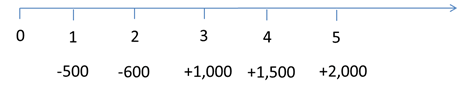# Present Value and Future Value of Uneven Cash Flows

We have looked at the PV/FV calculations for single sums of money and for annuities in which all the cash flows are equal. However, there may be an investment where the cash flows are not equal. We will now look at how to calculate the PV and FV of such an uneven series of cash flows.

Look at the following cash flows:Assuming an interest rate of 8%, we will now calculate the present value and future value of this uneven series of cash flows.

 Calculator Usage: Future value To calculate the future value of this series of cash flows, we will need to treat each cash flow as an independent cash flow and calculate its future value. We will adopt the procedure that we used to calculate the future value of a single cash flow.The following calculations are demonstrated using BA II Plus calculator.FV1: PV = -500, N = 4, I/Y = 8. CPT > FV = -$680.244FV2: PV = -600, N = 3, I/Y = 8. CPT > FV = -$755.827FV3: PV = +1,000, N = 2, I/Y = 8. CPT > FV = +$1,166.400FV4: PV = +1,500, N = 1, I/Y = 8. CPT > FV = +$1,620FV5: PV = +2,000, N = 0, I/Y = 8. CPT > FV = +$2,000Future Value of cash flows = Sum of all Future Values =$3350.328
 Calculator Usage: Present value To calculate the future value of this series of cash flows, we will need to treat each cash flow as an independent cash flow and calculate its future value. We will adopt the procedure that we used to calculate the present value of a single cash flow.PV1: FV = -500, N = 1, I/Y = 8. CPT > PV = -$462.963PV2: FV = -600, N = 2, I/Y = 8. CPT > PV = -$514.403PV3: FV = +1,000, N = 3, I/Y = 8. CPT > PV = +$793.832PV4: FV = +1,500, N = 4, I/Y = 8. CPT > PV = +$1,102.545PV5: FV = +2,000, N = 5, I/Y = 8. CPT > PV = +$1,361.166Future Value of cash flows = Sum of all Future Values =$2280.177

The present value of the uneven series of cash flows can also be calculated using the Cash Flow (CF) key and NPV function.